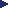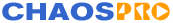HOMEFeaturesGalleryDownloadDocumentationResourcesMailing ListsProgramsFeature VotingWishlistTheoryBifurcationJulia/MandelD-SystemsLyapunovIFSL-SystemsPlasmaTutorialsFormula DBContactRelease 4.0### Bifurcation Diagrams

Well, most likely you already heard something about fractals called 'Bifurcation diagrams' or 'Verhulst diagrams'. If not, well, now you've heard something...

Perhaps you've even seen such a fractal. It does not look very aesthetic, so most people do not experiment with it. It makes much more fun creating beautiful images not caring about mathematical sense. If you agree to those people then there is no need to read on.

But if you don't agree with them, read on and try to understand the sense behind these diagrams.

Bifurcation diagram or Verhulst diagram?

Sometimes you will read about bifurcation diagrams, sometimes about Verhulst diagrams, do these two names mean just the same or are there differences? Well, as far as I know 'Bifurcation diagram' seems to be a general statement referring to a dynamic system which has bifurcations, whereas 'Verhulst diagram' most of the time simply references the model examined by Mr. Verhulst, namely y=a*x*(1-x) ...but I guess he examined many models.

The Model of Verhulst

Lets examine the model of Verhulst, an example of a bifurcation diagram. It describes how a population of animals (e.g. insects, hares, or something like that) grows from one generation to the next. This model is one of the most simple ones, and additionally it is very interesting and important (for science) so that very much has been written about it in almost every kind of magazines.

The model looks just like follows:

(1)    xn+1=a * xn * ( 1-xn )

with 0<=xn<=1 and 0<=a<=4

The biological meaning of xn is the relative amount of animals in the n-th generation, where 1 specifies the maximal amount of animals possible and 0 no animals at all. The index n specifies the time which goes by in small steps. The parameter 'a' is a measure for the population growth. If a<1 then the population will die out.

Now how can we work with this model? Lets start with an example: Lets set x=0.1, a=1.5, n=0, then lets apply the model to these values iteratively:

 `n` `population (xn)` `0` `0.1` `1` `a*x0*(1-x0) = 1.5*0.1*(1-0.1) = 0.1350` `2` `a*x1*(1-x1) = 1.5*0.1350*(1-0.1350) = 0.1752` `3` `0.2167` `4` `0.2546` `5` `0.2847` `6` `0.3055` `7` `0.3182` `8` `0.3254` `9` `0.3292` `10` `0.3313` `...` `...` `50` `0.3333`

In this example xn goes to 1/3 for larger n.

If we repeat this example with a=2.5, then again xn goes to 1/3 for large n. But if we examine x0, x1, ..., xn using a = 3.2, then we will see that the sequence of xn does not converge to 1/3 as before, but rather jumps between two values. A more close examination of xn shows us, that there can happen much more strange things, if we increase 'a' by small steps. It can happen that (xn)n>=0 jumps between m points. These m points are called m-cyclus. Or to say it in other words: We have an attractor consisting of m points. This attractor can even be a much more complicated construct, it need not consist of m discrete points, it can also consist of infinitely many points, a real strange attractor. In such a case we really can say that xn is attacted by the chaos.

In the model of Verhulst there exists an attractor for every value of 'a' between 0 and 4 and one can ask how the attractor depends on 'a', if one increases 'a' in small steps. It seems as if the attractor stays the same for some time, but then suddenly changes. This phenomenon is known as 'bifurcation' (and that's how these fractals got their name). One can create images showing these bifurcations, so called bifurcation diagrams.

Lets examine the Verhulst model for different intervals:

1st case: 0<a<=1

In this case x converges to 0, and this is clear, because 'a' is our growth rate, less than 1 ==> dying

2nd case: 1<a<=2

Here now the population quickly reaches a balance situation, the population is growing or shrinking (depending on the initial value of x) in a monotone way towards the attractor.

3rd case: 2<a<=3

Here still balance occurs, but the successive values of 'x' converge in an oscillating way to the balance-point and not in a monotone way.

Now let 'a' be grater than 3 e.g. a=3.1

`x1=0.3x2=0.651x3=0.704x4=0.646x5=...`

If you continue calculating values, then you'll recognize, that 'x' oscillates between two values, 0.557 and 0.764. So here we don't have a balance, the population is oscillating between these two values.

If you then take a bigger 'a', but less than 3.449489, then always the population oscillates between 2 values.

Starting with a=3.449489 something happens: A so called 'period-doubling' occurs, that means, 'x' suddenly oscillates between 4 values instead of 2. At a=3.5441 this 4-cyclus changes to an 8-cyclus. All these values, at which the cyclus lenght doubles, are called 'bifurcation nodes'. This 8-cyclus mutates to a 16-cyclus, then to a 32-cyclus, etc., upto a specific value: a=3.569946

Now it happens:

The whole attractor gets chaotic, that means 'x' oscillates randomly between an infinite amount of unconnected values, here now the attractor is not a cyclus with a fixed length any more, but rather a one dimensional fractal. In this range from 3.569946 upto 4 there are a few 'windows', e.g. at a=3.83, where a 3-cyclus dominates, which mutates to a 6-cyclus, then to a 12-cyclus, a 24-cyclus, etc.

'Windows' like that are spread over the range upto 4.

That's all for now, strange things can happen with such easy models.

Below you can see a small interface which lets you examine the bifurcation diagram of the formula xn+1=a * xn * ( 1-xn ).
Simply click onto the image: The text field below will display the generated series of xn+1=a * xn * ( 1-xn ) according to the position along the x-axis (which is the growth rate, i.e. the parameter a in the formula).

 Number of Iterations to perform: Growth rate (click on image to select): Initial Population:

Last update on Nov 26 2002.